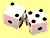Now suppose you want to generate a Monte Carlo Simulation of a fair coin with probability about 0.5 to get head and 0.5 to get tail, you can use the one of following formulas

Formula 1: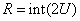, or

Formula 2: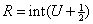Random number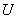that unformly distributed is generated according to previous page . To put this formula in action, I have made a worksheet in MS Excel. You may download it here . The explanation of how to use the worksheet is given below .

### How the formulas work?

Because random numberis in the range of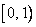, then using the first method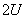will produce a range of zero to a maximum of almost reached 2. Since function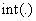will round the number down to the nearest integer, it will map range of 0 to 1 into 0 and range of 1 to almost 2 to 1.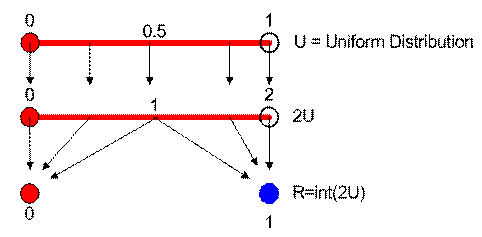Using the second method,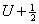maps the value offrom range ofinto range of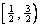. Thefunction will round down all values below 1 into 0 and round down all values between 1 and 1.5 into 1.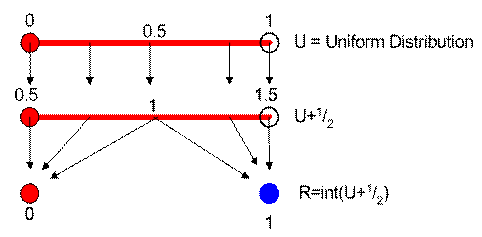The mean is 0.5 while the expected variance is 0.25

### How to use the worksheet?

Worksheet companion of this fair coins tutorial can be downloaded here.

The sample worksheet uses the two methods above to compute fair coins. Every time you press F9, it generates one sampling experiment of Monte Carlo Simulation. If you delete the word "STOP", every time you press F9, you are not only making one sample of numerical simulation; you will add the statistics of the sampling to all samples results. In this way, actually obtain full simple program of Monte Carlo Simulation.

To use the worksheet fully, delete the word "STOP" and press F9 repeatedly. If you don't delete the STOP, the simulation will still work but the result of all experiments are simply copies of the current experiment. Write any letter or number on that Blue cell to reset the counter.

The formula for mean and variance are recursive. You may see more explanation about this recursive formula in my tutorial on Recursive Statistics . If you are still confuse on how to use iteration in MS Excel, please read my tutorial on MS Excel Iteration .

Preferable reference for this tutorial is

Teknomo, Kardi. Monte Carlo Simulation Tutorial. http://people.revoledu.com/kardi/ tutoria/Simulation/